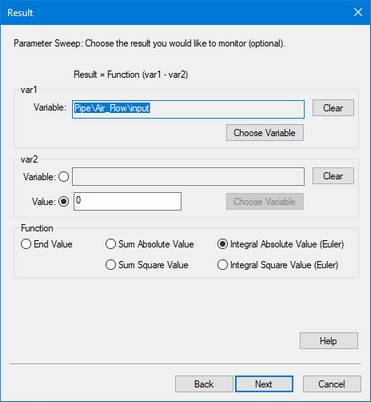﻿ 20-sim webhelp > Toolboxes > Time Domain Toolbox > Cost Function

# Cost Function

In the Multiple Run Wizard, the Result window allows you to define a result (the Cost Function) that will be used during simulations (Optimization, Curve Fitting) and displayed after the simulations runs (Parameter sweep, Optimization, Curve Fitting, Sensitivity, Monte Carlo, Variation Analysis, External DLL).The result can be a function of one or two variables. Instead of the second variable an (offset) value can be entered.

## Items

 • Choose Variable: Select this button to select a model variable
 • Clear: Use this button the remove a chosen model variable.
 • Function:
 • End value: The value at the end of the simulation run.
 • Sum Absolute Value: The sum of all absolute values during a simulation run.
 • Sum Square Value: The sum of all values squared during a simulation run.
 • Integral Absolute Value: The integral of all absolute values during a simulation run (uses Euler integration).
 • Integral Square Value: The integral of all values squared during a simulation run (uses Euler integration).

## User Defined Cost Function

You can easily use your own Cost Function for the result variable:

 1 Create your own cost function in the model.
 2 Use the Choose button to select as var1 the output of your function.
 3 Set var2 to a zero value.
 4 Choose End Value to prevent further operations on your function.